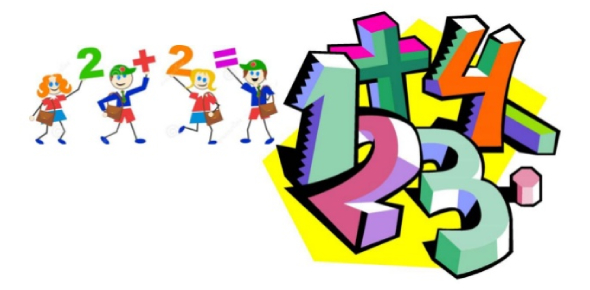# Addition And Subtraction Quiz: MCQ Exam!

10 Questions | Total Attempts: 1947SettingsAs a guardian of a grade one student, you understand that having them see how fun math is will alienate the chances of them having a negative attitude towards it. Help the young one understand how to tackle addition and subtraction math problems by taking the quiz below. Remember to put them in words they will understand.

• 1.
• A.

9

• B.

12

• C.

10

• D.

11

• 2.
What is the sum of 6 + 3 ?
• A.

5

• B.

9

• C.

10

• D.

11

• 3.
How many Fish are there?
• A.

6

• B.

8

• C.

7

• D.

9

• 4.
Use the number line to solve 9 - 4 = ?
• A.

4

• B.

3

• C.

5

• D.

2

• 5.
6 + 6 = ?
• A.

10

• B.

11

• C.

12

• D.

13

• 6.
10 +3 = ?
• A.

12

• B.

14

• C.

13

• D.

11

• 7.
9 + 6 = ?
• A.

13

• B.

11

• C.

15

• D.

14

• 8.
• A.

1

• B.

4

• C.

3

• D.

2

• 9.
6 + 7 =?
• A.

14

• B.

13

• C.

12

• D.

11

• 10.
10 - 8 = ?
• A.

4

• B.

5

• C.

2

• D.

1

Related TopicsBack to top